1. /
2. CBSE
3. /
4. Class 06
5. /
6. Mathematics
7. /
8. NCERT Solutions for Class...

# NCERT Solutions for Class 6 Maths Exercise 10.1

NCERT solutions for Maths Mensuration## NCERT Solutions for Class 6 Maths Mensuration

###### Question 1.Find the perimeter of each of the following figures: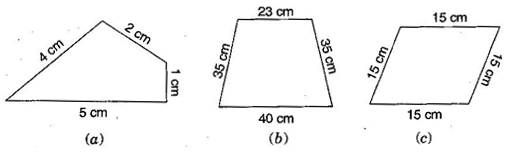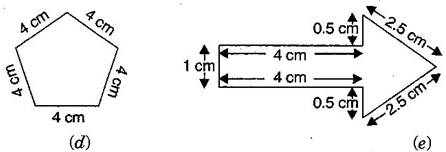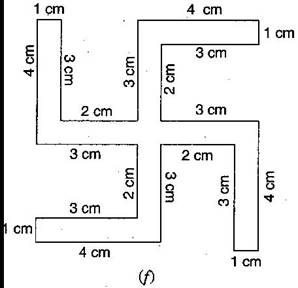(a) Perimeter = Sum of all the sides

= 4 cm + 2 cm + 1 cm + 5 cm = 12 cm

(b) Perimeter = Sum of all the sides

= 23 cm + 35 cm + 40 cm + 35 cm = 133 cm

(c)Perimeter = Sum of all the sides

= 15 cm + 15 cm + 15 cm + 15 cm = 60 cm

(d)Perimeter = Sum of all the sides

= 4 cm + 4 cm + 4 cm + 4 cm + 4 cm = 20 cm

(e)Perimeter = Sum of all the sides

1 cm + 4 cm + 0.5 cm + 2.5 cm + 2.5 cm + 0.5 cm + 4 cm = 15 cm

(f)Perimeter = Sum of all the sides

= 4 cm + 1 cm + 3 cm + 2 cm + 3 cm + 4 cm + 1 cm + 3 cm + 2 cm + 3 cm + 4 cm

1 cm + 3 cm + 2 cm + 3 cm + 4 cm + 1 cm + 3 cm + 2 cm + 3 cm

= 52 cm

NCERT Solutions for Class 6 Maths Exercise 10.1

###### Question 2.The lid of a rectangular box of sides 40 cm by 10 cm is sealed all round with tape. What is the length of the tape required?

Answer: Total length of tape required = Perimeter of rectangle

= 2 ( length + breadth)

= 2 ( 40 + 10)

= 2 x 50

= 100 cm = 1 m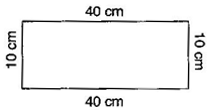Thus, the total length of tape required is 100 cm or 1 m.

###### Question 3. A table-top measures 2 m 25 cm by 1 m 50 cm. What is the perimeter of the table-top?

Answer: Length of table top = 2 m 25 cm = 2.25 m

Breadth of table top = 1 m 50 cm = 1.50 m

Perimeter of table top = 2 x (length + breadth)

= 2 x (2.25 + 1.50)

= 2 x 3.75 = 7.50 m

Thus, perimeter of table top is 7.5 m.

NCERT Solutions for Class 6 Maths Exercise 10.1

###### Question 4.What is the length of the wooden strip required to frame a photograph of length and breadth 32 cm and 21 cm respectively?

Length of wooden strip = Perimeter of photograph

Perimeter of photograph = 2 x (length + breadth)

= 2 (32 + 21)

= 2 x 53 cm = 106 cm

Thus, the length of the wooden strip required is equal to 106 cm.

###### Question 5.A rectangular piece of land measures 0.7 km by 0.5 km. Each side is to be fenced with 4 rows of wires. What is the length of the wire needed?

Answer: Since the 4 rows of wires are needed. Therefore the total length of wires is equal to 4 times the perimeter of rectangle.

Perimeter of field = 2 x (length + breadth) = 2 x (0.7 + 0.5) = 2 x 1.2 = 2.4 km

= 2.4 x 1000 m = 2400 m

Thus, the length of wire = 4 x 2400 = 9600 m = 9.6 m

NCERT Solutions for Class 6 Maths Exercise 10.1

###### Question 6.Find the perimeter of each of the following shapes:

(a)A triangle of sides 3 cm, 4 cm and 5 cm.

(b)An equilateral triangle of side 9 cm.

(c)An isosceles triangle with equal sides 8 cm each and third side 6 cm.

(a) Perimeter of {tex}\Delta {\text{ABC}}{/tex} = AB + BC + CA

= 3 cm + 5 cm = 4 cm

= 12 cm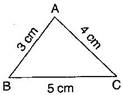(b) Perimeter of equilateral ABC = 3 x side

= 3 x 9 cm

= 27 cm(c) Perimeter of {tex}\Delta {\text{ABC}}{/tex} = AB + BC + CA

= 8 cm + 6 cm + 8 cm

= 22 cm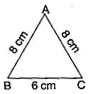NCERT Solutions for Class 6 Maths Exercise 10.1

###### Question 7.Find the perimeter of a triangle with sides measuring 10 cm, 14 cm and 15 cm.

Perimeter of triangle = Sum of all three sides

= 10 cm + 14 cm + 15 cm

= 39 cm

Thus, perimeter of triangle is 39 cm.

###### Question 8.Find the perimeter of a regular hexagon with each side measuring 8 cm.

Perimeter of Hexagon = 6 x length of one side

= 6 x 8 m

= 48 m

Thus, the perimeter of hexagon is 48 m.

NCERT Solutions for Class 6 Maths Exercise 10.1

###### Question 9.Find the side of the square whose perimeter is 20 m.

Answer: Perimeter of square = 4 x side

{tex} \Rightarrow {/tex} 20 = 4 x side

{tex} \Rightarrow {/tex} side = {tex}\frac{{20}}{4}{/tex} = 5 cm

Thus, the side of square is 5 cm.

###### Question 10.The perimeter of a regular pentagon is 100 cm. How long is its each side?

Perimeter of regular pentagon = 100 cm

{tex} \Rightarrow {/tex} 5 x side = 100 cm

{tex} \Rightarrow {/tex} side = {tex}\frac{{100}}{5}{/tex} = 20 cm

Thus, the side of regular pentagon is 20 cm.

NCERT Solutions for Class 6 Maths Exercise 10.1

###### Question 11.A piece of string is 30 cm long. What will be the length of each side if the string is used to form:

(a) a square

(b) an equilateral triangle

(c) a regular hexagon?

Length of string = Perimeter of each figure

(a)Perimeter of square = 30 cm

{tex} \Rightarrow {/tex} 4 x side = 30 cm

{tex} \Rightarrow {/tex} side = {tex}\frac{{30}}{4}{/tex} = 7.5 cm

Thus, the length of each side of square is 7.5 cm.

(b)Perimeter of equilateral triangle = 30 cm

{tex} \Rightarrow {/tex} 3 x side = 30 cm

{tex} \Rightarrow {/tex} side = {tex}\frac{{30}}{3}{/tex} = 10 cm

Thus, the length of each side of equilateral triangle is 10 cm.

(c)Perimeter of hexagon = 30 cm

{tex} \Rightarrow {/tex} 6 x side = 30 cm

{tex} \Rightarrow {/tex} side = {tex}\frac{{30}}{6}{/tex} = 5 cm

Thus, the side of each side of hexagon is 5 cm.

###### Question 12.Two sides of a triangle are 12 cm and 14 cm. The perimeter of the triangle is 36 cm. What is the third side?

Let the length of third side be {tex}x{/tex} cm.

Length of other two side are 12 cm and 14 cm.

Now, Perimeter of triangle = 36 cm

{tex} \Rightarrow {/tex} {tex}12 + 14 + x = 36{/tex}

{tex} \Rightarrow {/tex} {tex}26 + x = 36{/tex}

{tex} \Rightarrow {/tex} {tex}x = 36 – 26{/tex}

{tex} \Rightarrow {/tex} {tex}x = 10{/tex} cm

Thus, the length of third side is 10 cm.

NCERT Solutions for Class 6 Maths Exercise 10.1

###### Question 13.Find the cost of fencing a square park of side 250 m at the rate of ` 20 per meter.

Side of square = 250 m

Perimeter of square = 4 x side

= 4 x 250 = 1000 m

Since, cost of fencing of per meter = Rs. 20

Therefore, cost of fencing of 1000 meters = 20 x 1000 = = Rs. 20,000

###### Question 14.Find the cost of fencing a rectangular park of length 175 m and breadth 125 m at the rate of Rs. 12 per meter.

Length of rectangular park = 175 m

Breadth of rectangular park = 125 m

Perimeter of park = 2 x (length + breadth)

= 2 x (175 + 125)

= 2 x 300 = 600 m

Since, cost of fencing park per meter = = Rs. 12

Therefore, cost of fencing park of 600 m = 12 x 600 = Rs. 7,200

NCERT Solutions for Class 6 Maths Exercise 10.1

###### Question 15Sweety runs around a square park of side 75 m. Bulbul runs around a rectangular park with length of 60 m and breadth 45 m. Who covers less distance?

Distance covered by Sweety = Perimeter of square park

Perimeter of square = 4 x side

= 4 x 75 = 300 m

Thus, distance covered by Sweety is 300 m.

Now, distance covered by Bulbul = Perimeter of rectangular park

Perimeter of rectangular park = 2 x (length + breadth)

= 2 x (60 + 45)

= 2 x 105 = 210 m

Thus, Bulbul covers the distance of 210 m.

And Bulbul covers less distance.

###### Question 16.What is the perimeter of each of the following figures? What do you infer from the answer?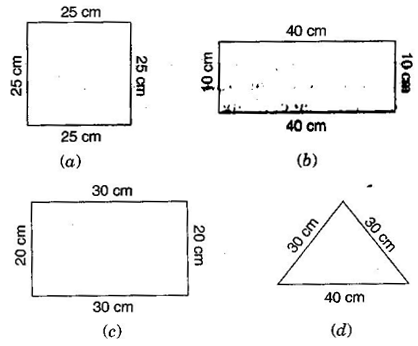(a) Perimeter of square = 4 x side

= 4 x 25 = 100 cm

(b) Perimeter of rectangle = 2 x (length + breadth)

= 2 x (40 + 10)

= 2 x 50 = 100 cm

(c) Perimeter of rectangle = 2 x (length + breadth)

= 2 x (30 + 20)

= 2 x 50 = 100 cm

(d)Perimeter of triangle = Sum of all sides

= 30 cm + 30 cm + 40 cm = 100 cm

Thus, all the figures have same perimeter.

NCERT Solutions for Class 6 Maths Exercise 10.1

###### Question 17.Avneet buys 9 square paving slabs, each with a side {tex}\frac{1}{2}{/tex} m. He lays them in the form of a square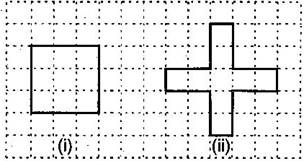(a)What is the perimeter of his arrangement?

(b)Shari does not like his arrangement. She gets him to lay them out like a cross. What is the perimeter of her arrangement?

(c)Which has greater perimeter?

(d)Avneet wonders, if there is a way of getting an even greater perimeter. Can you find a way of doing this? (The paving slabs must meet along complete edges, i.e., they cannot be broken.)

(a) 6 m

(b) 10 m

(c) Second arrangement has greater perimeter.

(d) Yes, if all the squares are arranged in row, the perimeter be 10 cm.

## NCERT Solutions for Class 6 Maths Exercise 10.1

NCERT Solutions Class 6 Maths PDF (Download) Free from myCBSEguide app and myCBSEguide website. Ncert solution class 6 Maths includes text book solutions from Class 6 Maths Book . NCERT Solutions for CBSE Class 6 Maths have total 14 chapters. 6 Maths NCERT Solutions in PDF for free Download on our website. Ncert Maths class 6 solutions PDF and Maths ncert class 6 PDF solutions with latest modifications and as per the latest CBSE syllabus are only available in myCBSEguide.

## CBSE app for Students

To download NCERT Solutions for Class 6 Maths, Social Science Computer Science, Home Science, Hindi English, Maths Science do check myCBSEguide app or website. myCBSEguide provides sample papers with solution, test papers for chapter-wise practice, NCERT solutions, NCERT Exemplar solutions, quick revision notes for ready reference, CBSE guess papers and CBSE important question papers. Sample Paper all are made available through the best app for CBSE students and myCBSEguide website.Test Generator

Create question paper PDF and online tests with your own name & logo in minutes.myCBSEguide

Question Bank, Mock Tests, Exam Papers, NCERT Solutions, Sample Papers, Notes

1. Excellent

2. Outstanding